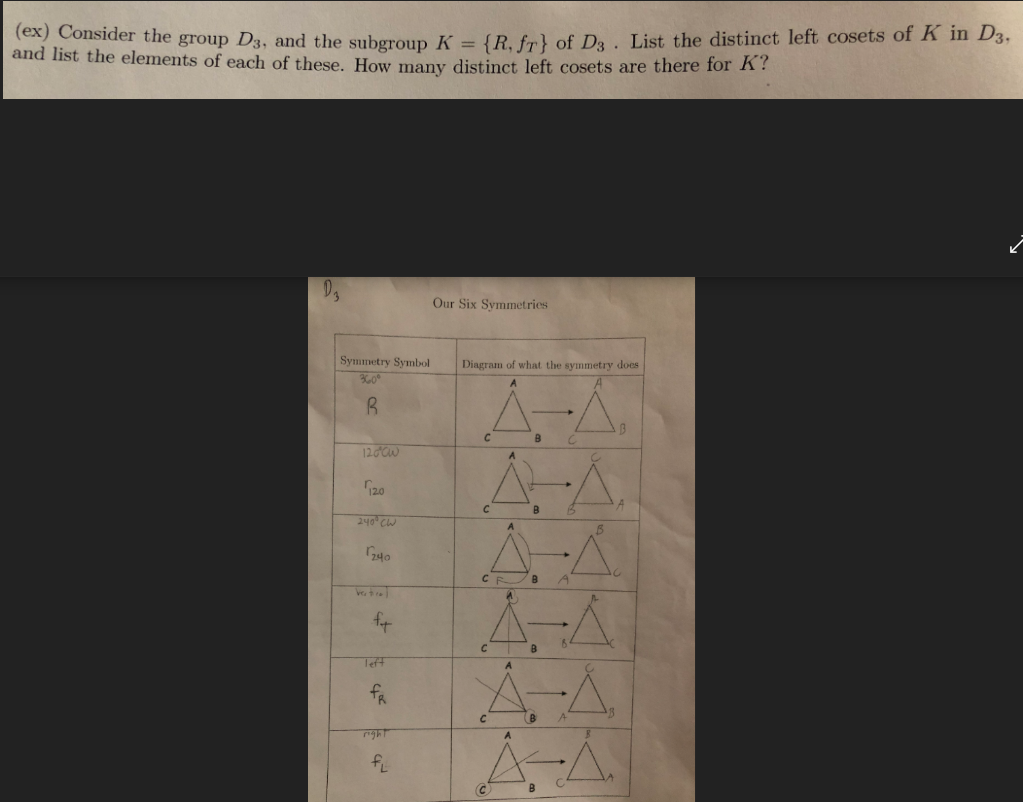# (ex) Consider the group Ds, and the subgroup K -R. fr of Da . List the distinct left cosets of K in D3.d list the elements of each of these. How many distinct left cosets are there for ?Our Six SymmetriesSymmetry Symbol Diagram of what the symmetry does120a0120240TR

Question
1 views

Abstract Algebra

The problem and D3 is given in the below picture.help_outlineImage Transcriptionclose(ex) Consider the group Ds, and the subgroup K -R. fr of Da . List the distinct left cosets of K in D3. d list the elements of each of these. How many distinct left cosets are there for ? Our Six Symmetries Symmetry Symbol Diagram of what the symmetry does 120a0 120 240 TR fullscreen
check_circle

Step 1
Step 2

The coset RK can be listed as follows.

Step 3

The coset r120K can be listed a...

### Want to see the full answer?

See Solution

#### Want to see this answer and more?

Solutions are written by subject experts who are available 24/7. Questions are typically answered within 1 hour.*

See Solution
*Response times may vary by subject and question.
Tagged in

### Math